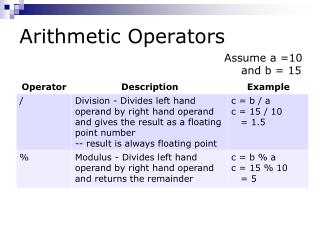Download PresentationArithmetic Operators

Arithmetic Operators - PowerPoint PPT Presentation

Arithmetic Operators. Assume a =10 and b = 15. Arithmetic Operators. Although frequently referred to as integer division , Python’s terminology of floor division is clearer. Modulus and Floor. Where would modulus be useful?I am the owner, or an agent authorized to act on behalf of the owner, of the copyrighted work described.
Download PresentationArithmetic Operators

Download Policy: Content on the Website is provided to you AS IS for your information and personal use and may not be sold / licensed / shared on other websites without getting consent from its author.While downloading, if for some reason you are not able to download a presentation, the publisher may have deleted the file from their server.

- - - - - - - - - - - - - - - - - - - - - - - - - - E N D - - - - - - - - - - - - - - - - - - - - - - - - - -
Presentation Transcript
1. Arithmetic Operators • Assume a =10 and b = 15

2. Arithmetic Operators • Although frequently referred to as integer division, Python’s terminology of floor division is clearer.

3. Modulus and Floor Where would modulus be useful? Modulus gives a remainder – what is left over. Where would floor be useful? Floor tells you how many times an amount can be divided evenly (with no remainder). Typical application: a quantity of items is divided into groups … how many groups will you have? How many items are left over?

4. Where would modulus/floor be useful? A packing company receives a skid containing 1300 packages of KD. These packages are repacked into bulk boxes for various vendors: e.g. Sam’s Club 12 packages/box Amazon 15 packages/box USA Food Store 35 packages/case. Write a program to prompt the user for the vendor’s name and the number of packages in the bulk container and calculate the number of boxes/cases of KD and the number of remaining packages given one skid.

5. Arithmetic Operators

6. Operator Precedence • From highest to lowest: • 1. calculations in brackets • 2. exponentiation (**) • 3. multiplication (*), division (/ or //), remainder (%) • 4. addition (+), subtraction (-) • If operators in same precedence level, execute left to right.

7. Calculation Examples 30/10 + 5 * 6/2 = 30/(10 + 5 * 6)/2 = 30/(10 + 5) * (6/2) =

8. Formulas to Equations